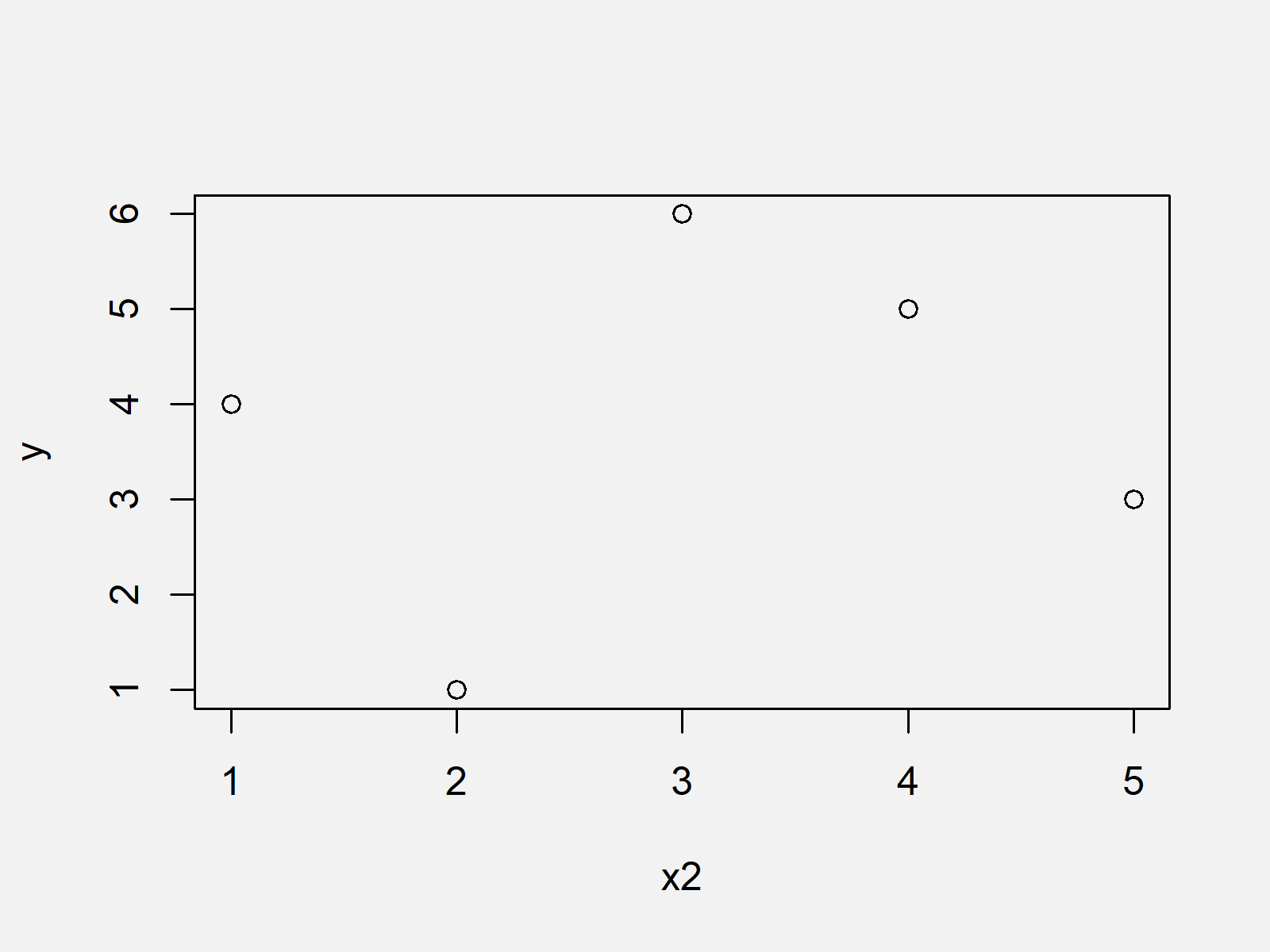# R Error in plot.window(…) : need finite ‘xlim’ values (2 Examples)

This article illustrates how to handle the plot error “need finite ‘xlim’ values” in R.

Let’s dive into it…

## Creation of Example Data

As a first step, we need to create some example data. First, we’ll create an x-vector:

```x <- rep(NA, 5)              # Create x vector
x                            # Print x vector
# NA NA NA NA NA```

Have a look at the previous output of the RStudio console. It shows that our x vector contains only NA values.

Let’s also create a y vector:

```y <- c(4, 1, 6, 5, 3)        # Create y vector
y                            # Print y vector
# 4 1 6 5 3```

Our y vector consists of different numeric values.

Let’s assume that we want to plot these data…

## Example 1: Reproduce the Error Message – need finite ‘xlim’ values

In this example, I’ll illustrate how to replicate the error message “need finite ‘xlim’ values” in R.

If we want to draw our previously created data to a plot, we might try to use the following R code:

```plot(x, y)                   # Try to draw plot
# Error in plot.window(...) : need finite 'xlim' values
# 1: In min(x) : no non-missing arguments to min; returning Inf
# 2: In max(x) : no non-missing arguments to max; returning -Inf```

As you can see, the previous syntax returned the error message “need finite ‘xlim’ values”.

That’s obviously no surprise. Our x vector contains only missing values and hence cannot be drawn to a plot.

So how can we solve this problem?

## Example 2: Avoid the Error Message – need finite ‘xlim’ values

In Example 2, I’ll show how to fix the problems with the error message “need finite ‘xlim’ values”.

First, we have to create an x vector that contains actual values:

`x2 <- 1:5                    # Creating proper x vector`

Now, we can use this vector and our previously created y vector to draw a plot:

`plot(x2, y)                  # Drawing plot`As shown in Figure 1, the previously shown syntax created a scatterplot in Base R.

## Video & Further Resources

Have a look at the following video of my YouTube channel. I illustrate the R programming code of this article in the video:

Please accept YouTube cookies to play this video. By accepting you will be accessing content from YouTube, a service provided by an external third party.If you accept this notice, your choice will be saved and the page will refresh.

In addition, you may want to read the other posts of my homepage. I have released numerous tutorials about topics such as labels and graphics in R:

At this point in the article you should know how to deal with the plotting error message “need finite ‘xlim’ values” in the R programming language.

Please note that a very similar error message could occur, in case all y-values are NA (i.e. “Error in plot.window(…) : need finite ‘ylim’ values”).

Don’t hesitate to tell me about it in the comments, in case you have any additional questions.

Subscribe to the Statistics Globe Newsletter